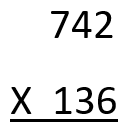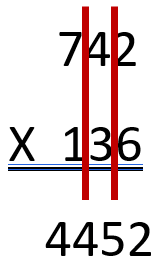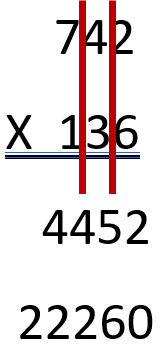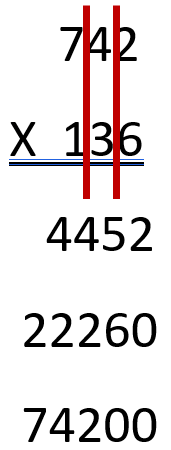# Math Worksheets Land

Math Worksheets For All Ages

# Math Worksheets Land

Math Worksheets For All Ages

# Long Multiplication Worksheets

We explore a strategy that can be applied to solving the product of larger values very quickly and smoothly. We explore two different approaches to solving these problems which are not always the easiest to process that quickly. For some people one method just clicks with them. Stick with the one that works best for you. This collection of worksheets will help students learn to multiply large numbers.

### Aligned Standard: Grade 4 Base Ten - 4.NBT.5

• Answer Keys - These are for all the unlocked materials above.

### Homework Sheets

We find the products of numbers and numbers mixed with a slight visual counting exercise.

• Homework 1 - If the result is more than 9, carry over the digit to the next place value.
• Visuals Homework 2 - We use images here. This is a different approach that may help a few students.
• Homework 3 - We have to divide the result by multiplicand.

### Practice Worksheets

The follow the same format as the previous series.

• Practice 1 - A blank slate to work off of. You have plenty of space to work with. Make sure to account for each place value.
• Visuals Practice 2 - Visuals to help you through it. You basically have multiple sets of those groups to work with.
• Practice 3 - Find the missing part of each multiplication problem.

### Math Skill Quizzes

Many teachers requested that I mixed visuals with numbers. So I did try to accommodate that in each quiz.

• Quiz 1 - Find the product of missing part of the problem.
• Quiz 2 - Fill in the quiz problems. What is not present in each of these problems?
• Quiz 3 - Some visuals can be found here to get you started off.

### How to Solve Long Multiplication Problems

Long multiplication problems involve finding the product between two numbers that consist of at least two and three digits. The basic strategy used to solve these types of problems is called either the column or grid method. You can also use the expanded strategy which works very well. We will explore all these different strategies in action. Take the following problem using all the different strategies: 742 x 136

## Column or Grid Method

Step 1: Stack the numbers one on top of the other. The larger value normally sits at the top.Step 2: To make it easier to organize, place each place value into a grid or column. Start multiplication from the right side and multiply each digit of the second from each digit of the number on the top. Multiply 6 by 2,4, and finally 7.Step 3: Proceeding from the right side, move to the second digit of the bottom number and multiply it by the above number. Remember to the placement of zero because it is the tens place.Step 4: Continue the process until you multiply the last digit, approaching from the right side of the bottom number with the number on top. You will need two ending zeroes because this is the hundreds place.Step 5: Find the sum of the numbers below the sum-line to find the answer. That would be 4,452 + 22,260 + 74,200 = 100,912

## Expanded Form Multiplication

When we use this strategy, we break one of the values into expanded form and multiply it in parts and then find the final sum to determine the overall product. It is very similar to the last strategy just with a slightly different orientation change.

Step 1: Working with the product of 742 x 136, we would break the lesser value into expanded form and re-write the problem as:

742 x 6 =

742 x 30 =

742 x 100 =

Step 2: Process each of those products.

742 x 6 = 4,452

742 x 30 = 22,260

742 x 100 = 74,200

Step 3: Find the sum of all those values to determine the end product. 4,452 + 22,260 + 74,200 = 100,912

Unlock all the answers, worksheets, homework, tests and more!
Save Tons of Time! Make My Life Easier Now

## Thanks and Don't Forget To Tell Your Friends!

I would appreciate everyone letting me know if you find any errors. I'm getting a little older these days and my eyes are going. Please contact me, to let me know. I'll fix it ASAP.